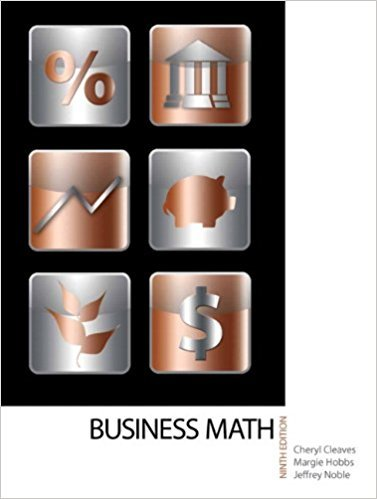×
×

# Solutions for Chapter 14-1: FUTURE VALUE OF AN ANNUITY## Full solutions for Business Math, | 9th Edition

ISBN: 9780135108178Solutions for Chapter 14-1: FUTURE VALUE OF AN ANNUITY

Solutions for Chapter 14-1
4 5 0 367 Reviews
14
2
##### ISBN: 9780135108178

This textbook survival guide was created for the textbook: Business Math, , edition: 9. This expansive textbook survival guide covers the following chapters and their solutions. Business Math, was written by and is associated to the ISBN: 9780135108178. Since 28 problems in chapter 14-1: FUTURE VALUE OF AN ANNUITY have been answered, more than 17286 students have viewed full step-by-step solutions from this chapter. Chapter 14-1: FUTURE VALUE OF AN ANNUITY includes 28 full step-by-step solutions.

Key Math Terms and definitions covered in this textbook
• Augmented matrix [A b].

Ax = b is solvable when b is in the column space of A; then [A b] has the same rank as A. Elimination on [A b] keeps equations correct.

• Basis for V.

Independent vectors VI, ... , v d whose linear combinations give each vector in V as v = CIVI + ... + CdVd. V has many bases, each basis gives unique c's. A vector space has many bases!

A sequence of steps (end of Chapter 9) to solve positive definite Ax = b by minimizing !x T Ax - x Tb over growing Krylov subspaces.

• Determinant IAI = det(A).

Defined by det I = 1, sign reversal for row exchange, and linearity in each row. Then IAI = 0 when A is singular. Also IABI = IAIIBI and

• Diagonal matrix D.

dij = 0 if i #- j. Block-diagonal: zero outside square blocks Du.

• Distributive Law

A(B + C) = AB + AC. Add then multiply, or mUltiply then add.

• Free columns of A.

Columns without pivots; these are combinations of earlier columns.

• Fundamental Theorem.

The nullspace N (A) and row space C (AT) are orthogonal complements in Rn(perpendicular from Ax = 0 with dimensions rand n - r). Applied to AT, the column space C(A) is the orthogonal complement of N(AT) in Rm.

• Inverse matrix A-I.

Square matrix with A-I A = I and AA-l = I. No inverse if det A = 0 and rank(A) < n and Ax = 0 for a nonzero vector x. The inverses of AB and AT are B-1 A-I and (A-I)T. Cofactor formula (A-l)ij = Cji! detA.

• Kirchhoff's Laws.

Current Law: net current (in minus out) is zero at each node. Voltage Law: Potential differences (voltage drops) add to zero around any closed loop.

• Krylov subspace Kj(A, b).

The subspace spanned by b, Ab, ... , Aj-Ib. Numerical methods approximate A -I b by x j with residual b - Ax j in this subspace. A good basis for K j requires only multiplication by A at each step.

• Markov matrix M.

All mij > 0 and each column sum is 1. Largest eigenvalue A = 1. If mij > 0, the columns of Mk approach the steady state eigenvector M s = s > O.

• Minimal polynomial of A.

The lowest degree polynomial with meA) = zero matrix. This is peA) = det(A - AI) if no eigenvalues are repeated; always meA) divides peA).

• Pivot columns of A.

Columns that contain pivots after row reduction. These are not combinations of earlier columns. The pivot columns are a basis for the column space.

• Polar decomposition A = Q H.

Orthogonal Q times positive (semi)definite H.

• Projection matrix P onto subspace S.

Projection p = P b is the closest point to b in S, error e = b - Pb is perpendicularto S. p 2 = P = pT, eigenvalues are 1 or 0, eigenvectors are in S or S...L. If columns of A = basis for S then P = A (AT A) -1 AT.

• Right inverse A+.

If A has full row rank m, then A+ = AT(AAT)-l has AA+ = 1m.

• Solvable system Ax = b.

The right side b is in the column space of A.

• Unitary matrix UH = U T = U-I.

Orthonormal columns (complex analog of Q).

• Volume of box.

The rows (or the columns) of A generate a box with volume I det(A) I.

×